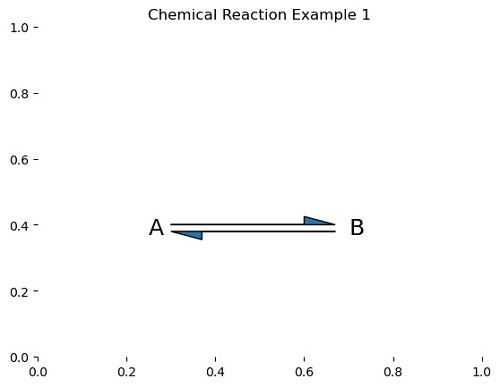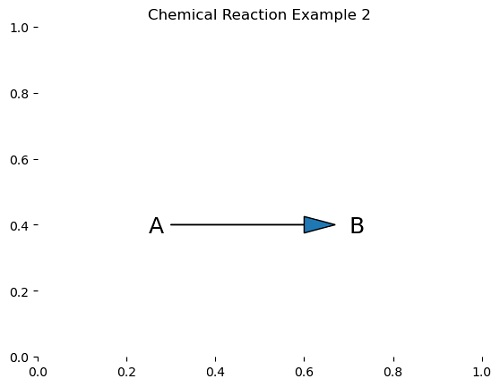# Python | Drawing Chemical Reaction using Arrow (Matplotlib Arrow Example)

Here, we are going to learn how to draw chemical reaction using arrow (matplotlib arrow example)?
Submitted by Anuj Singh, on July 31, 2020

Arrows are very frequently used in drawing chemical reactions. Therefore, in this article, we are going to illustrate two different examples of how to draw a chemical reaction in python using matplotlib.

Example 1 is showing a chemical reaction with equilibrium between reactant A and product B.Example 2 is showing a chemical reaction where reactant A is converted into product B.## Python code for drawing chemical reaction using arrow

```import matplotlib.pyplot as plt

plt.figure()
plt.text(0.25, 0.37, 'A', fontsize=18)

plt.text(0.7, 0.37, 'B', fontsize=18)

plt.title('Chemical Reaction Example 1')
plt.box()
plt.show()

plt.figure()
plt.text(0.25, 0.375, 'A', fontsize=18)
plt.text(0.7, 0.375, 'B', fontsize=18)
plt.title('Chemical Reaction Example 2')
plt.box()
plt.show()
```

Output:

```Output is as figure
```